# Formula Mass

## Atom and Molecule of Class 9

The formula mass of an ionic compound is the relative mass of its formula unit as compared with the mass of a carbon atom (carbon - 12) taken as 12 units.

e.g. To find the formula mass of potassium carbonate (K2CO3).

Formula mass of K2CO3 = mass of 2 K atoms + Mass of 1 C atom + Mass of 3 O atoms

= (2 × 39) + (1 × 12) + (3 × 16)

= 78 + 12 + 48

= 138 u .

Hence, the formula mass of K2CO3 is 138 u.

## WRITING A CHEMICAL FORMULA:

Before we learn how to write a chemical formula we should know what is a chemical formula? A chemical formula may be defined as the “Symbolic representation of its composition” or in other words,

“A chemical formula of a molecular compound represents the actual number of atoms present in one molecule of the compound”.

For example: H2O is the chemical formula of water, NH3 is the chemical formula of ammonia.

In other words, the formula of a compound tells us the “kind of atoms” as well as “the number of atoms” of various elements present in one molecule of the compound.

“Chemical formula of an ionic compound represents the cations and anions present in the structure of the compound”.

For example: Na+Cl represents that sodium chloride contains Na+ and Cl ions in the ratio of 1:1.

To understand how to write the chemical formula of different compounds, we need to learn the symbols and combining capacity of the elements.

(a) Formula of Elements:

The chemical formula of an element is a representation of the composition of its molecule in which symbol represents the element and subscript represents, how many atoms are present in one molecule.

e.g. One molecule of hydrogen element contains two atoms of hydrogen, therefore, the formula of hydrogen is H2. It should, however, be noted that 2H represents two separate atoms of hydrogen, while H2 represents one molecule of hydrogen.

(b) Formula of Comp​ounds:

The chemical formula of a compound is representation of the composition of its molecule in which symbol represents, which elements are present and the subscript shows us how many atoms of each element are present in one molecule of a compound. e.g. One molecule of water contains 2 atoms of hydrogen and 1 atom of oxygen. Hence, the formula of water is H2O.

### CONCEPT OF VALENCY:

Valency can be defined as the combining capacity of that particular element.

To find out how the atoms of an element will combine with the atom (s) of another element to form a compound, valency can be used.

For example  valency of oxygen is 2, this means that one atom of oxygen can combine with 2 atoms of hydrogen or in other words we can say that valency of hydrogen is one so 2 atoms of hydrogen can combine with one atom of oxygen to form water molecule.

The concept of valency can be understood more easily by the following examples. The valency of the atom of an element can be considered as hands of that atom.

We know that human beings have two hands and an octopus has eight. If one octopus has to catch hold of a few people in such a way that both arms of the entire human and all the eight arms of octopus are locked, how many humans according to you an octopus can hold?

If we represent the octopus with O and humans with H than we can write a formula for this combination as OH4 where 4 is the subscript which indicates the number of humans held by the octopus.

As molecular compounds are formed by the combination of non-metal atoms, the valencies of some of them are as follows:

### Valencies of some common non-metal elements

Element

Symbol

Valency

Element

Symbol

Valency

Hydrogen

H

1

Oxygen

O

2

Fluorine

F

1

Sulphur

S

2, 4, 6

Chlorine

Cl

1

Nitrogen

N

3, 5

Bromine

Br

1

Phosphorus

P

3, 5

Iodine

I

1

Carbon

C

4

 Valency of some elements may be variable depends on the number of electrons present in outermost shell.

For writing the chemical formula of an ionic compound, valency of an ion can be defined as – “the units of positive or negative charge present on the ion.”

For example: Na+ ion has one unit positive charge

Ca2+ ion has two unit positive charges

Cl ion has one unit negative charge

SOion has two unit negative charges

Depending upon whether the ions has 1, 2, 3 or 4 unit charge (positive or negative), they are called monovalent, divalent, trivalent and tetravalent ions respectively.

Below is given a table showing names and symbols of some ion

### Names and symbols of some ions

Valency

Name of ion

Symbol

Non-metallic element

Symbol

Polyatomic ions

Symbol

1.

Sodium

Na+

Hydrogen

H+

Ammonium

NH4+

Potassium

K+

Hydride

H

Hydroxide

OH

Silver

Ag+

Chloride

Cl

Nitrate

NO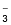Copper (I)*

Cu+

Bromide

Br

Hydrogen

carbonate

HCOIodide

I

2.

Magnesium

Mg2+

Oxide

O2

Carbonate

CO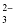Calcium

Ca2+

Sulphide

S2

Sulphite

SOZinc

Zn2+

Sulphate

SO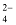Iron (II)*

Fe2+

Copper (II)*

Cu2+

3.

Aluminium

Al3+

Nitride

N3

Phosphate

PO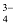Iron (III)*

Fe3+

From the above table, it is seen that some elements show a number of valencies (called variable valencies). For example, copper shows a valency of 1 as well as 2, iron shows a valency of 2 as well as 3. These valencies are sometimes shown by Roman numeral in a bracket after the symbol of the element, e.g., Cu (I), Cu (II), Fe (II), Fe (III) etc. Some non-metal atoms also show variable valency.

Related Topics

### RULES FOR WRITING THE CHEMICAL FORMULAE

• Chemical formulas represent compounds (2 or more elements chemically combined).
• Valence numbers are used to determine how many of each element is used in the compound.
• Compounds are composed of a positive half and a negative half.
• The positive half is always written first.
• The total of the valence numbers must equal zero.
•  Subscripts (small numbers to lower right of chemical symbol) represent the number of each molecule of the element in each compound.
• The subscript of 1 is never written in a chemical formula
• Add subscripts, when needed, to make the oxidation numbers total ZERO
• Use the criss-cross method to write the chemical formula
• Reduce subscripts if they can be divisible by the SAME number  Ca2O2 = CaO

### Formulae of Simple Compounds:

The simplest compounds, which are made up of two different elements are called binary compounds.

By applying the above mentioned rules, the chemical formulae of some simple molecular compounds can be written as follow:

e.g. Steps for writing the formula of Hydrogen chloride:

•  Elements present areHydrogen and chlorine
• Symbols of the elementsH Cl
• Valency of the elements1 1
• Cross-over of the valency: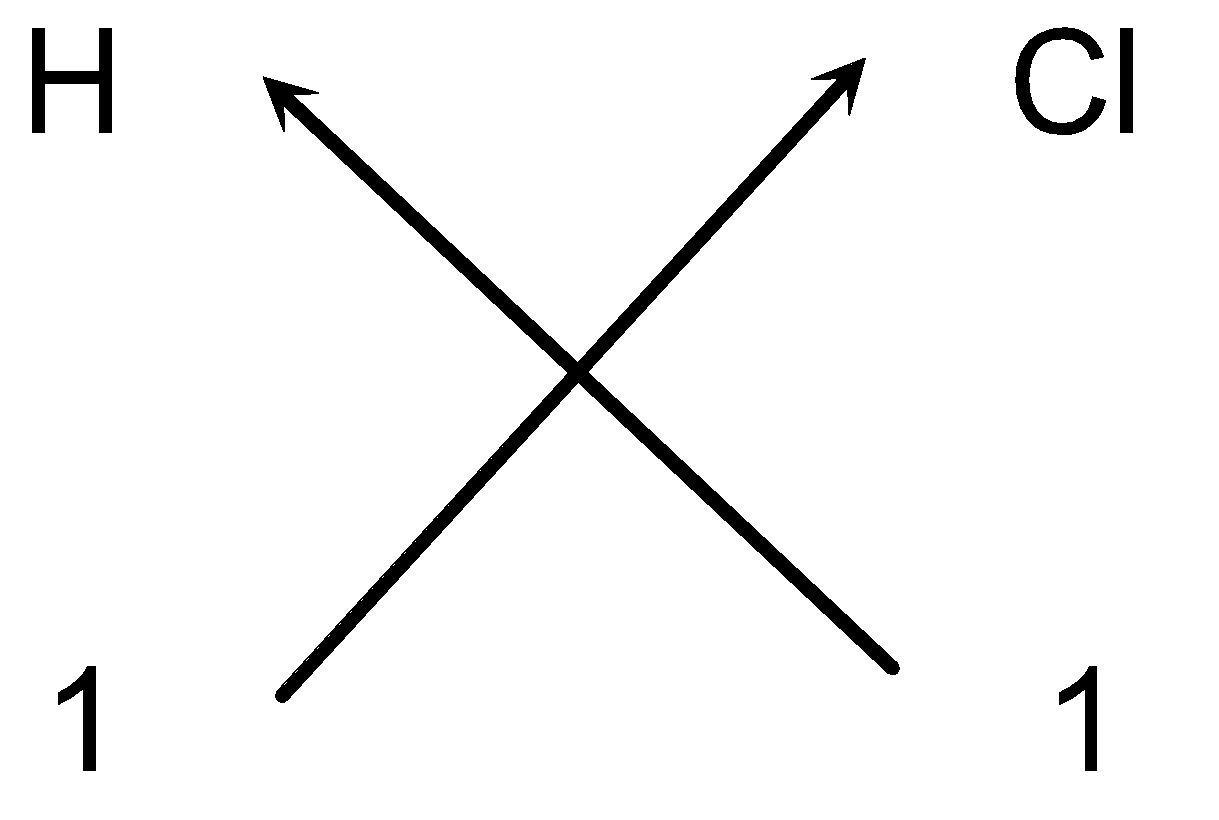• We stated that one appearing on the lower right hand and left hand side of the symbol is omitted. So the formula of the compound would be HCl.

e.g. Steps for writing the formula of Methane :

• Elements present areCarbon    Hydrogen
• Symbol of the elementsC H
• Valency of the elements4 1
• Cross-over of the valency: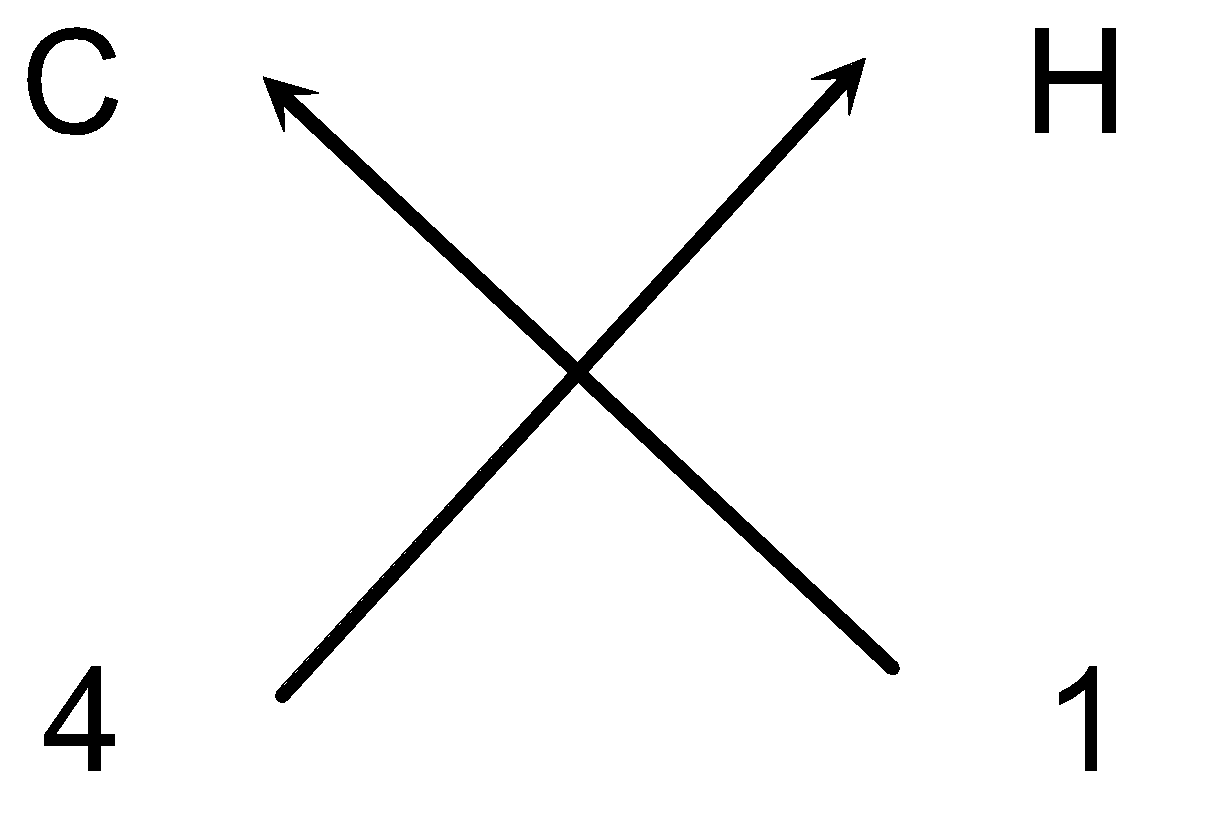• So the chemical formula of the methane can be written as CH4 (As we omit one appearing on the lower right hand side of carbon element).

e.g. Steps for writing the chemical formula of Carbon dioxide

• Elements present areCarbon    Oxygen
• Symbols of the elementsC O
•  Valency of the elements4 2
• Dividing by common factor: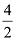= 2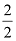= 1
• Cross-over of the valency: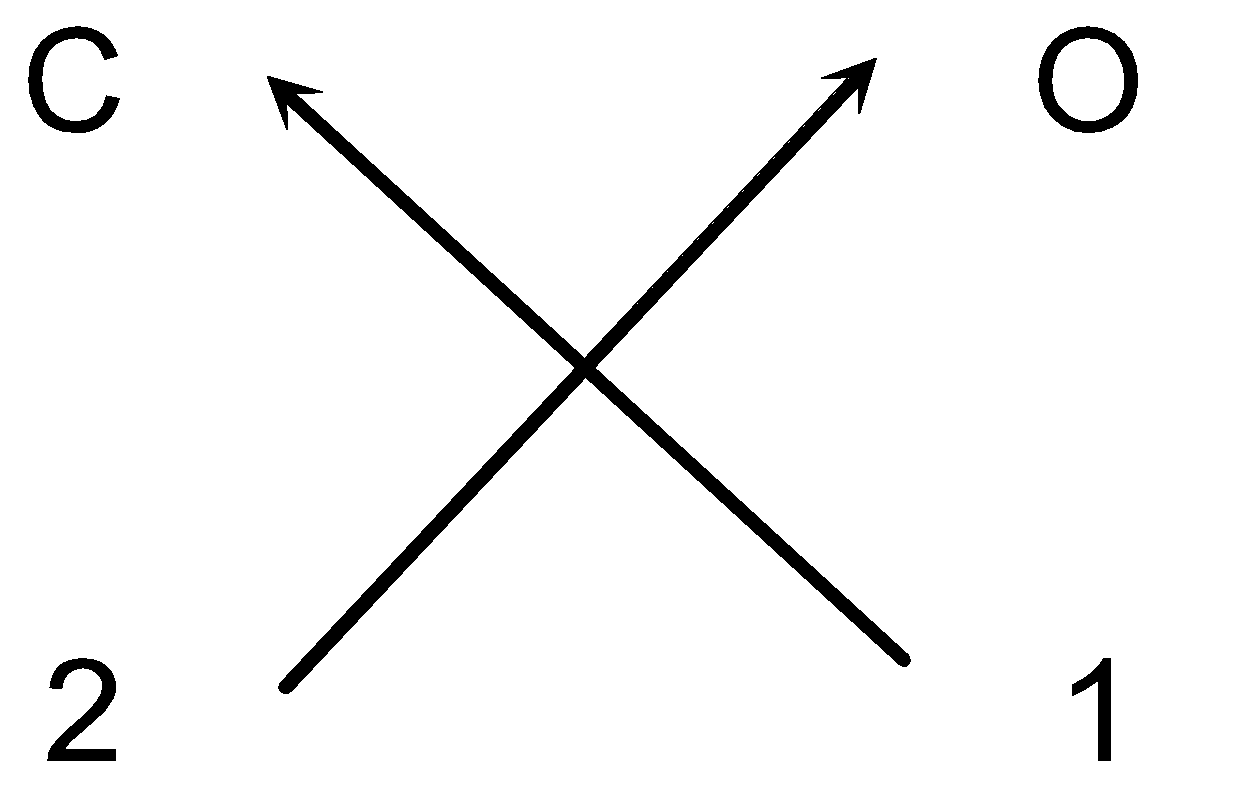• So the chemical formula of carbon dioxide can be written as CO2 (as we omit one appearing on the lower right hand side of carbon element).

Chemical formulae of some simple ionic compound

e.g. Steps for writing the chemical formula of Sodium chloride

• Elements present in the compoundSodium Chlorine
• Symbols of the elements:Na Cl
• Charge on the ions+1 −1
•  Valency of the elements:1 1
• Cross-over of the valency: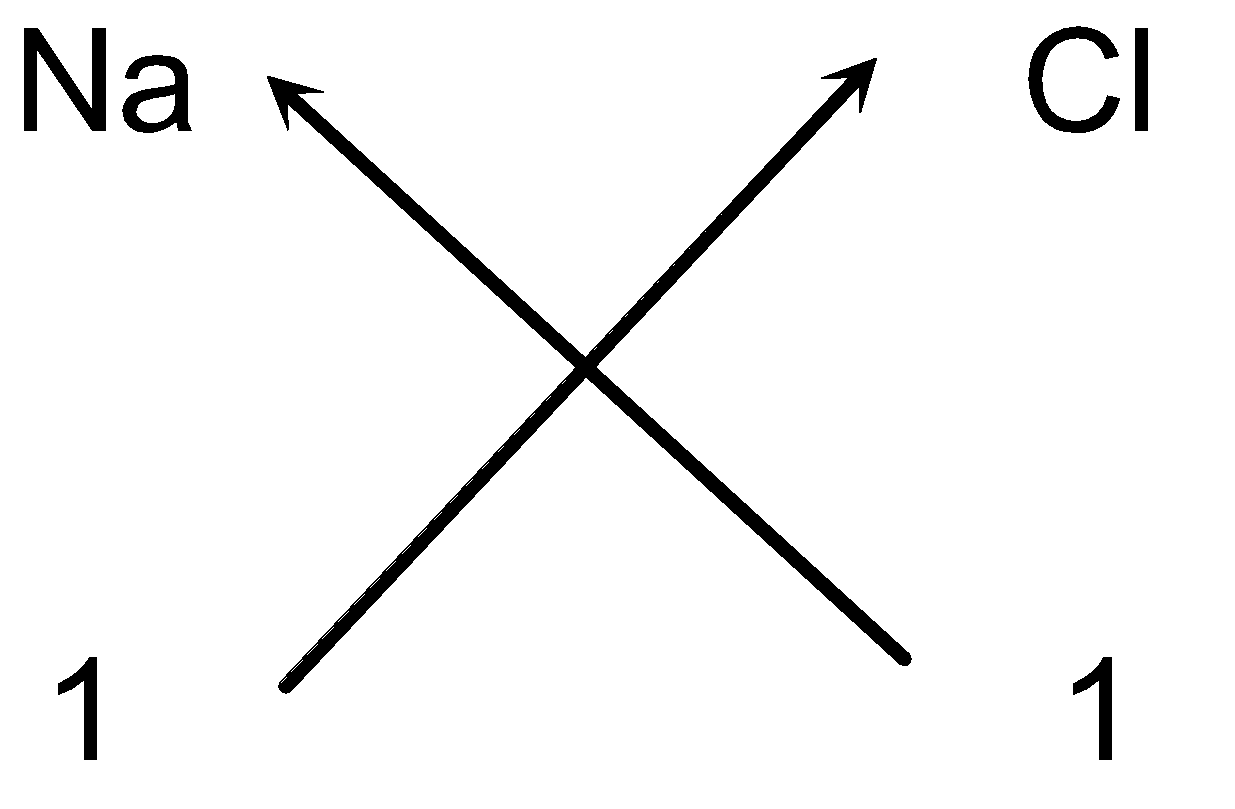• So the chemical formula of sodium chloride can be written as NaCl (As we omit 1 appearing on the lower right hand side of Na and Cl atoms and + and – sign of the charges of ions).

e.g. Steps for writing the chemical formula of Aluminium oxide

• Elements present in the compound:Aluminium Oxygen
• Symbols of the elements:Al O
• Charge on the ions+3 −2
• Valency of the elements:3 2
• Cross-over of the valency: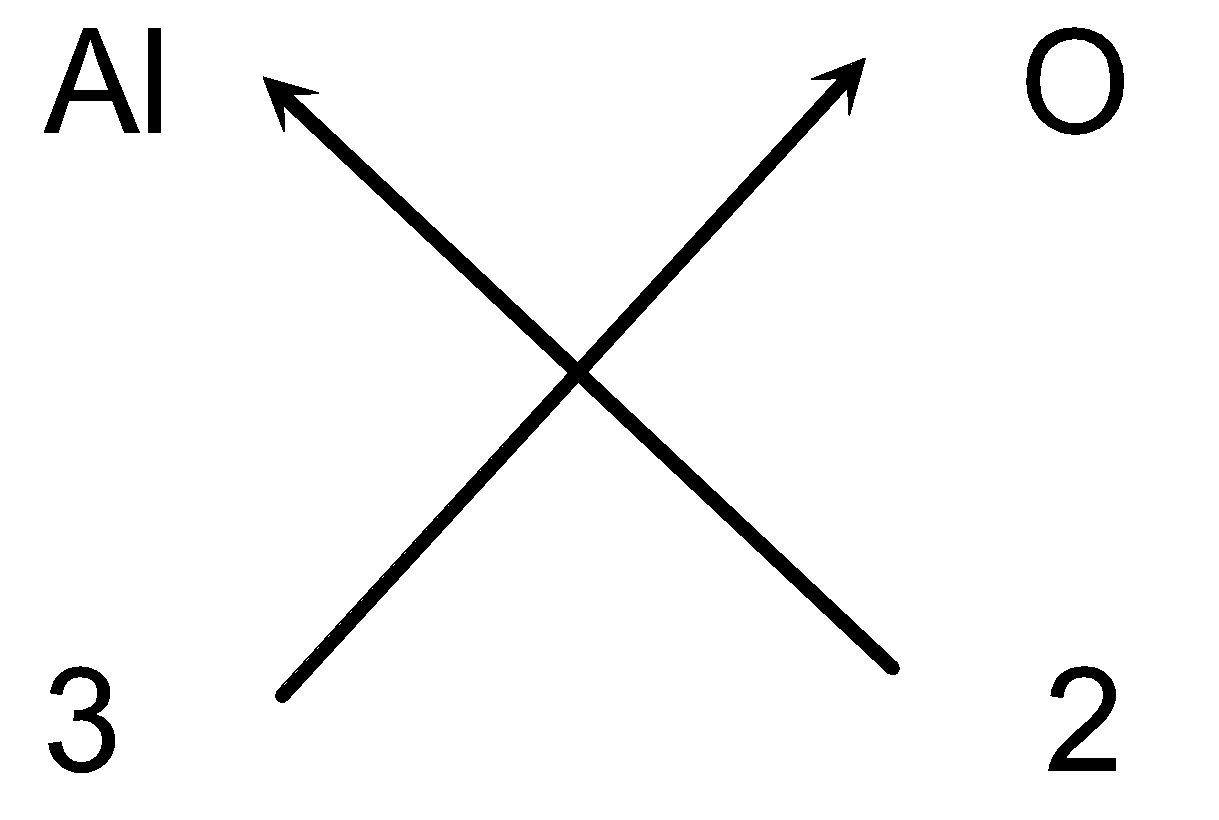• So the chemical formula of aluminium oxide can be written as Al2O3.

Chemical formulae of compounds containing polyatomic ions:

While writing the chemical formulae of compounds containing polyatomic ions, same rules will apply except that the formula of polyatomic ion is written in brackets.

e.g. Steps for writing the formula of Potassium nitrate

• Symbols of the ions:K (NO3)
• Charge on the ions:1+ 1−
• Cross-over of the valency: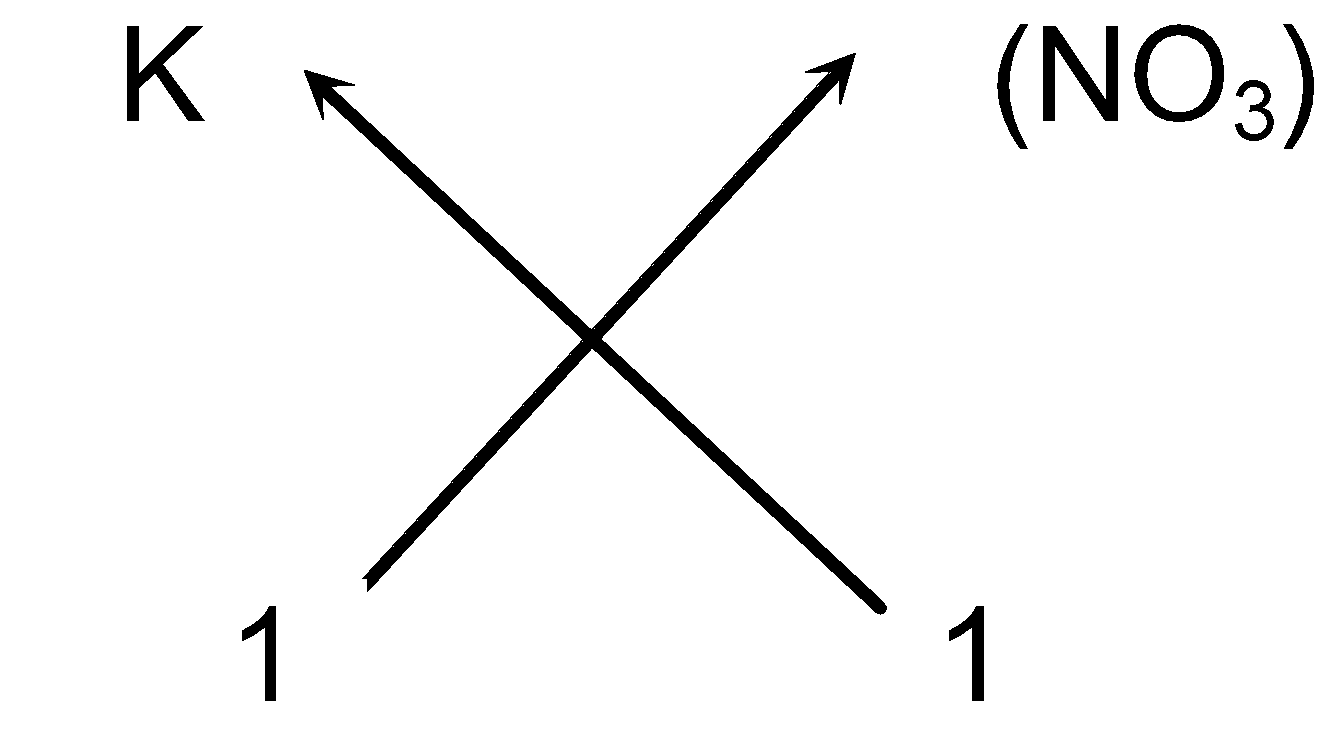(v) So the chemical formula of Potassium nitrate can be written as KNO3 (As we omit one appearing on the right hand side of the ions).

e.g. Steps for writing the chemical formula of Calcium nitrate

• Symbols of the ions:Ca (NO3)
•  Charge on the ions:2+ 1−
•  Valency of the ions:2 1
•  Cross-over of the valency: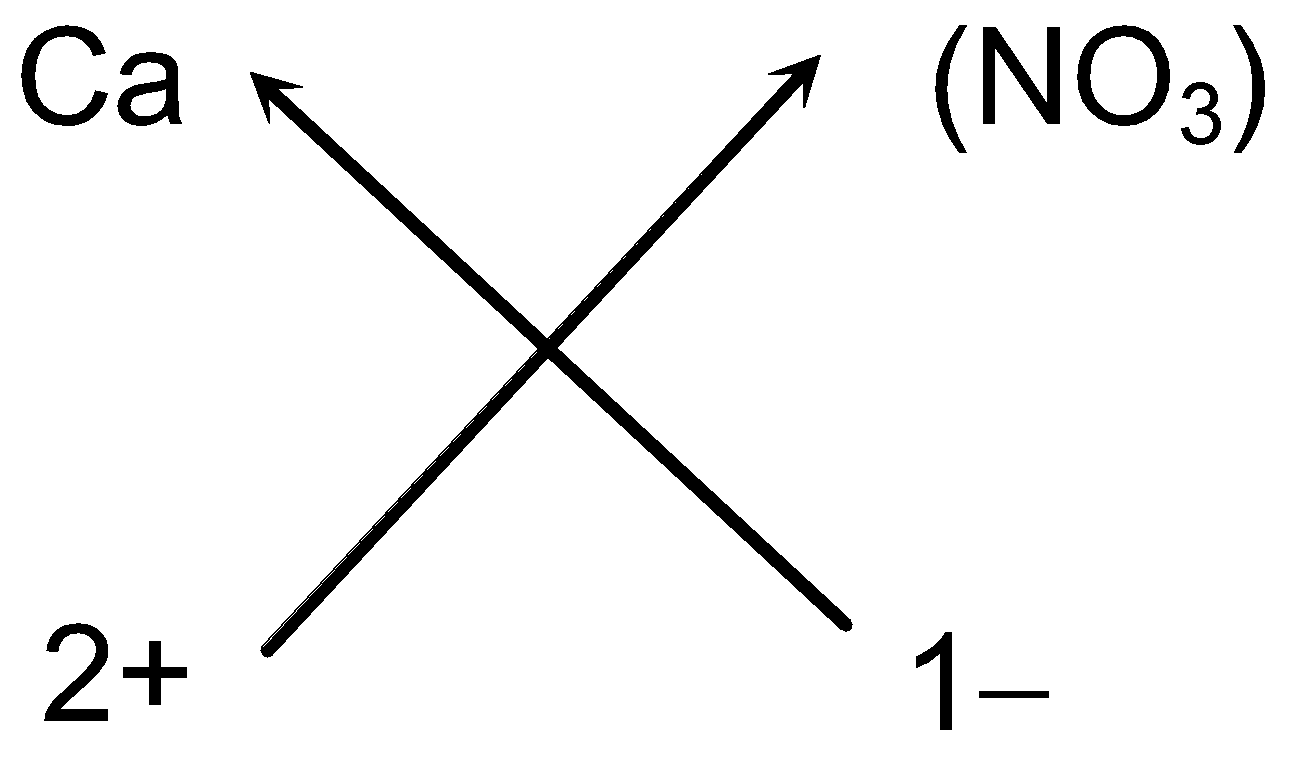• So the chemical formula of Calcium nitrate can be written as Ca (NO3)2 (As we omit one appearing on the right hand side of the Ca ions).

e.g. Steps for writing the chemical formula of Ammonium sulphate

• Symbols of the ions present:(NH4) (SO4)
• Charge on the ions:1+ 2−
• Valency of the ions:1 2
• Cross-over of the valency: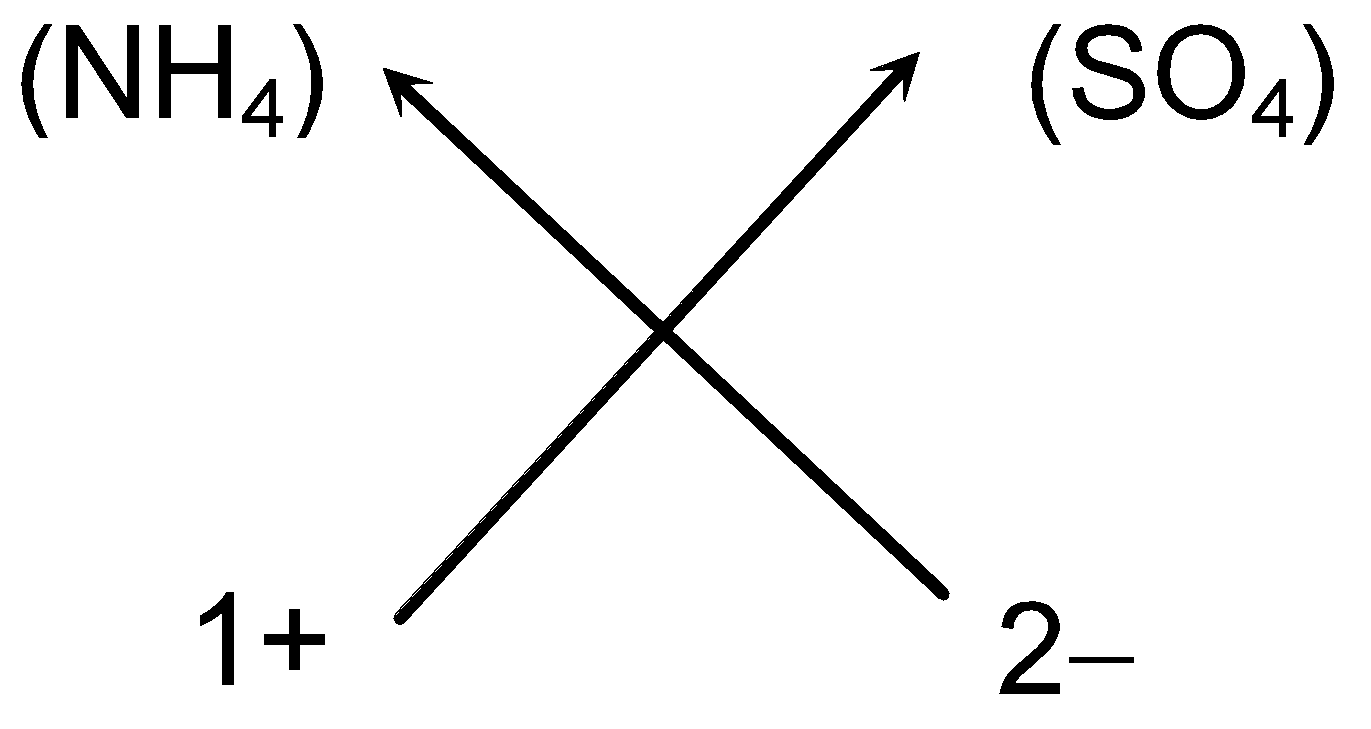(v) So we can write the chemical formula of ammonium sulphate as (NH4)2SO4 (As we omit one appearing on the right hand side of sulphate ion).

Examples:

1. An element E is trivalent. Write the chemical formula of its (i) chloride (ii) oxide (iii) sulphide.

Solution: (a) Steps for writing the chemical formula of Chloride of trivalent element E.

(i) Elements present: Element Chloride

(ii) Symbols: E Cl

(iii) Valency: 3 1

(iv) Cross-over of valency: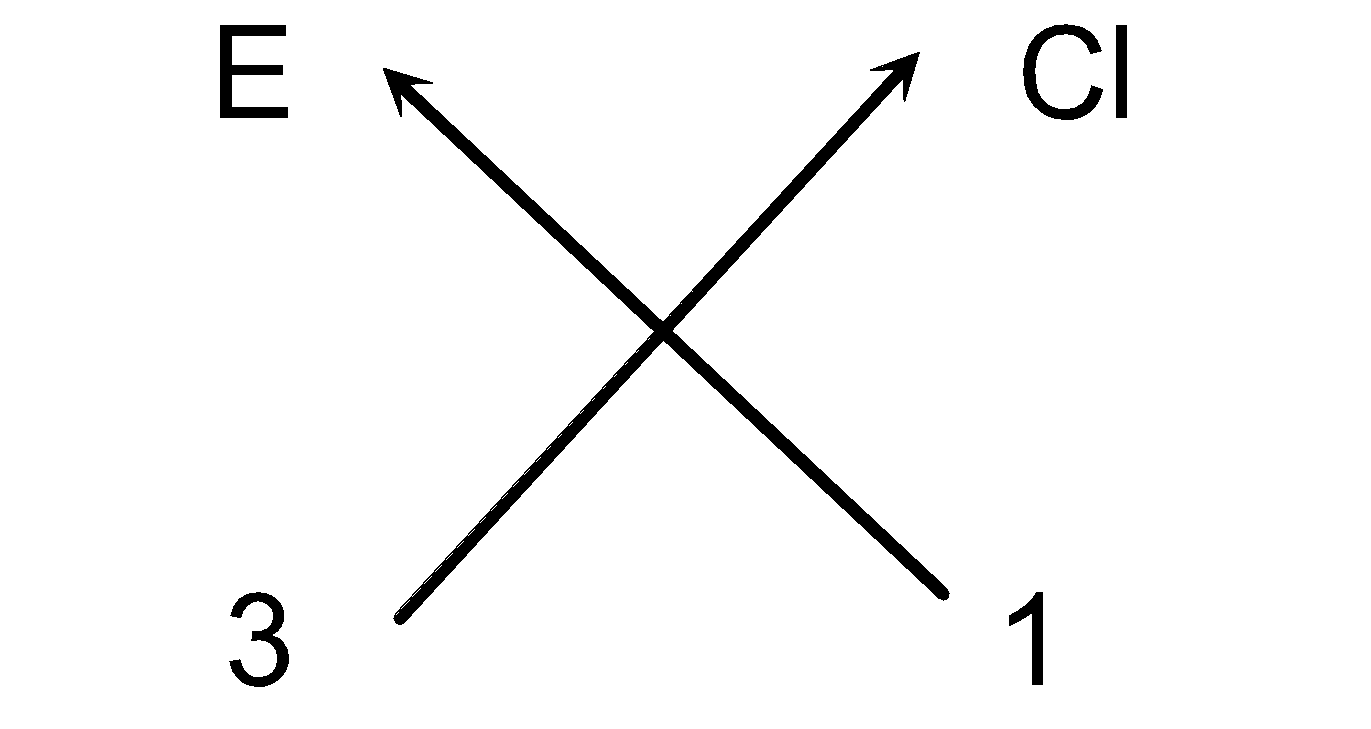(v) Thus, we can write the chemical formula as ECl3.

(a) Steps for writing the chemical formula of Oxide of trivalent element E.

(i) Elements present: Element Oxygen

(ii) Symbols: E O

(iii) Valency: 3 2

(iv) Cross-over of valency: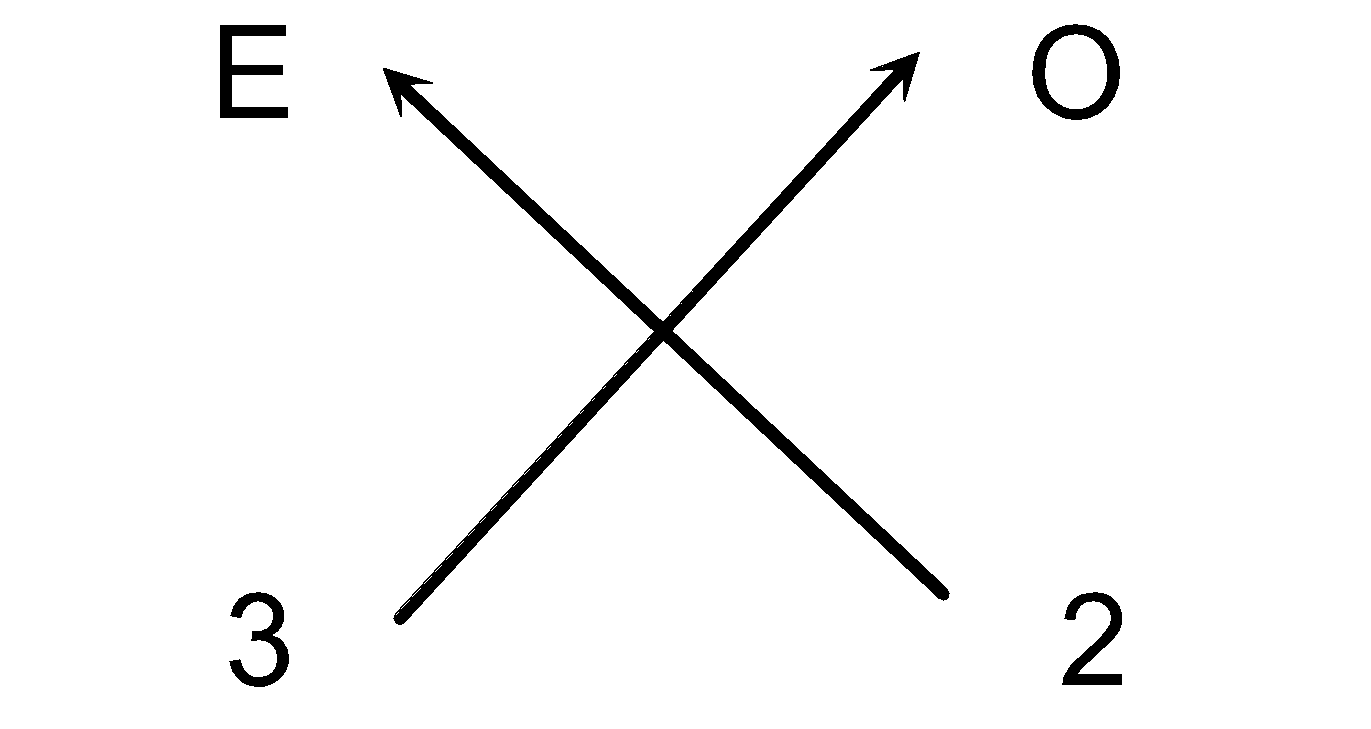(v) Thus we can write the chemical formula as E2O3.

(c) Steps for writing the chemical formula of Sulphide of trivalent element E.

•  Elements present: Element Sulphur
• Symbols: E S
•  Valency: 3 2
•  Cross-over of valency: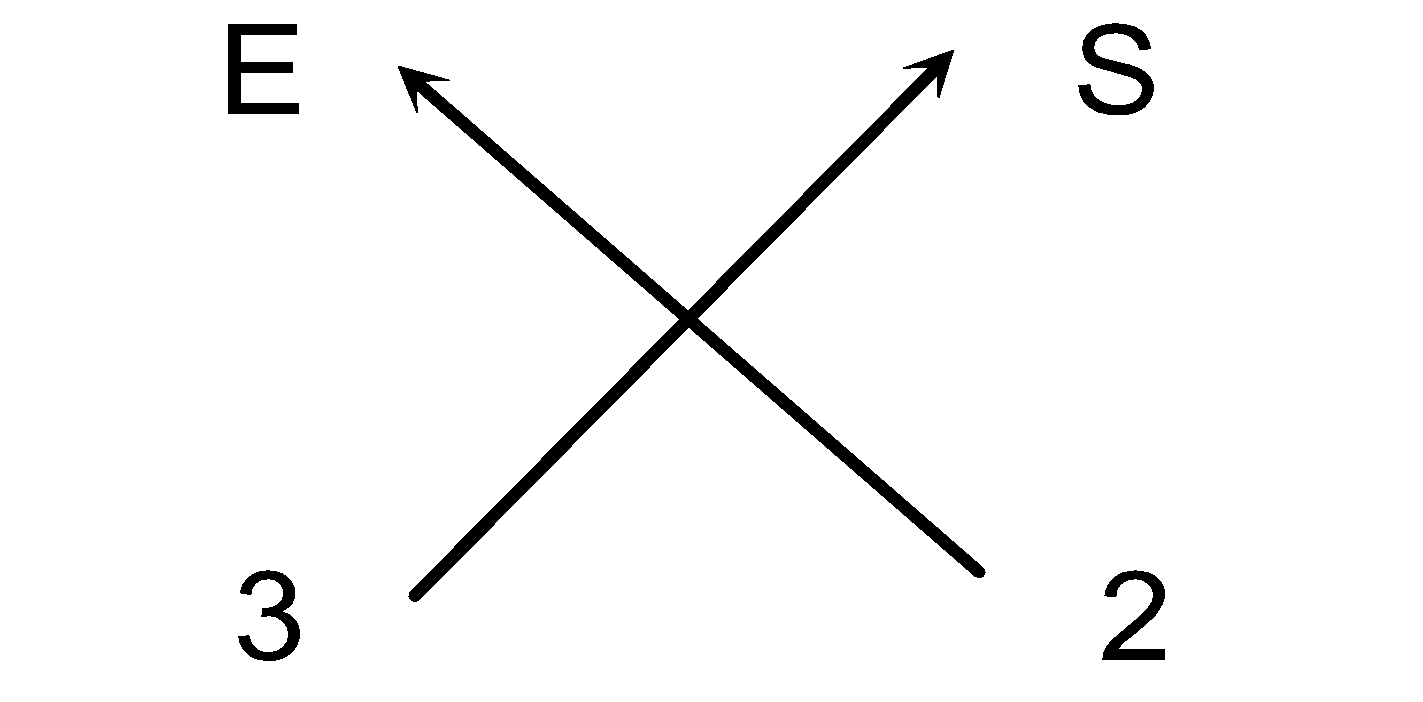(v) Thus we can write the chemical formula as E2S3

2. An element M forms the oxide M2O3. What will be the formula of its phosphate?

Solution:  (i) In M2O3 total charge on 2 oxide ion = 3 x (−2) = −6

(ii) As the compound M2O3 is neutral so the charge on two metal atoms should be = +6

∴ Charge on one metal atom = + 6/2 = + 3

i.e. valency of metal atom = 3

Steps for writing the formula of metal M phosphate.

• Symbols of the elements/ion present: M (PO4)
• Charge: 3+ 3−
• Valency: 3 3
•  Dividing by the common factor= 1= 1
• Cross-over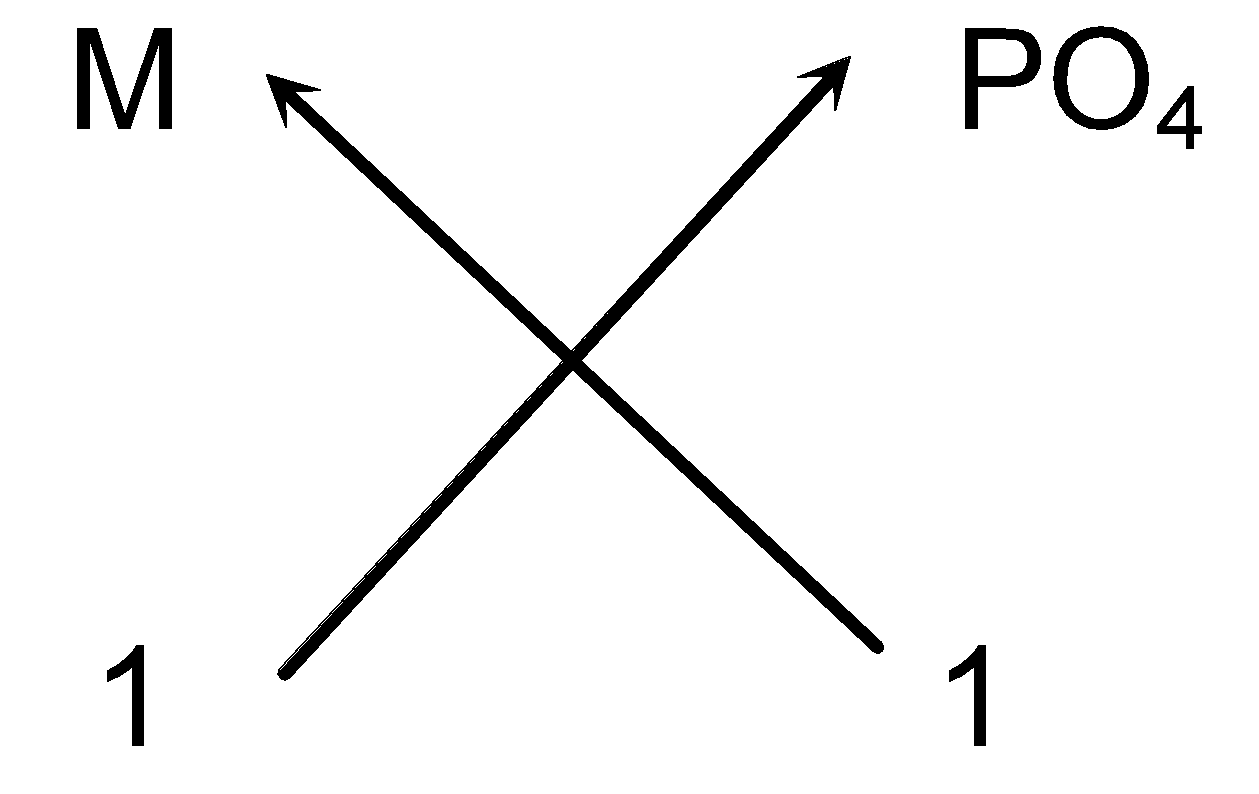• Thus we can write the chemical formula as MPO4

To score More in your class 9 refer NCERT solutions for class 9